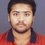# Factor Group

Can anyone help me in this question :-

Prove or disprove: If $H$ is a normal subgroup of $G$ such that $H$ and $G/H$ are abelian, then $G$ is abelian.

I don't need any counter example. I need a proper proof.Note by Kushal Bose
4 years, 1 month ago

This discussion board is a place to discuss our Daily Challenges and the math and science related to those challenges. Explanations are more than just a solution — they should explain the steps and thinking strategies that you used to obtain the solution. Comments should further the discussion of math and science.

When posting on Brilliant:

• Use the emojis to react to an explanation, whether you're congratulating a job well done , or just really confused .
• Ask specific questions about the challenge or the steps in somebody's explanation. Well-posed questions can add a lot to the discussion, but posting "I don't understand!" doesn't help anyone.
• Try to contribute something new to the discussion, whether it is an extension, generalization or other idea related to the challenge.

MarkdownAppears as
*italics* or _italics_ italics
**bold** or __bold__ bold
- bulleted- list
• bulleted
• list
1. numbered2. list
1. numbered
2. list
Note: you must add a full line of space before and after lists for them to show up correctly
paragraph 1paragraph 2

paragraph 1

paragraph 2

[example link](https://brilliant.org)example link
> This is a quote
This is a quote
    # I indented these lines
# 4 spaces, and now they show
# up as a code block.

print "hello world"
# I indented these lines
# 4 spaces, and now they show
# up as a code block.

print "hello world"
MathAppears as
Remember to wrap math in $$ ... $$ or $ ... $ to ensure proper formatting.
2 \times 3 $2 \times 3$
2^{34} $2^{34}$
a_{i-1} $a_{i-1}$
\frac{2}{3} $\frac{2}{3}$
\sqrt{2} $\sqrt{2}$
\sum_{i=1}^3 $\sum_{i=1}^3$
\sin \theta $\sin \theta$
\boxed{123} $\boxed{123}$

Sort by:

I don't need any counter example. I need a proper proof.

Well, it turns out that giving a counter example is a proper proof.

Taking $G=S_3$ and $H=A_3$ we see that the hypothesis is satisfied though $G$ isn't abelian.

- 4 years ago

- 4 years ago

I don't need any counter example. I need a proper proof.

Unfortunately, false statements cannot be proven in a consistent system.

Of course they can be!

- 4 years ago

How can they?

I meant they can be proven to be false.

- 4 years ago

Yep.

But I suppose there are statements which cannot be proven or disproven.

Much like this one, which has no proof.

Yes. In any consistent system there exist statements which can't be proved or disproved. To disprove here means to prove the negation.

- 4 years ago

Yes. How does that make you feel?

@Deeparaj Bhat Check this out

- 4 years, 1 month ago